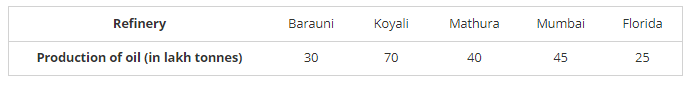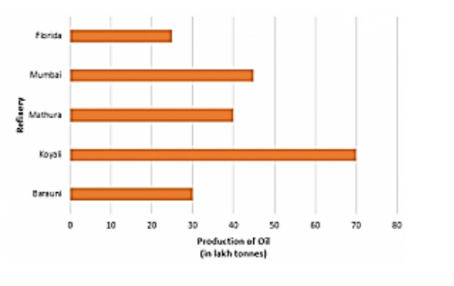# The production of oil (in lakh tonnes) in some of the refineries in India during 1982 was given below:

Question:

The production of oil (in lakh tonnes) in some of the refineries in India during 1982 was given below:Construct a bar graph to represent the above data so that the bars are drawn horizontally.

Solution:

To represent the given data by a vertical bar graph, we first draw horizontal and vertical axes. Let u consider that the vertical and horizontal axes represent the refineries and the production of oil in la] tonnes respectively. We have to draw 5 bars of different lengths given in the table.

At first, we mark 5 points in the vertical axis at equal distances and erect rectangles of the same wk at these points. The lengths of the rectangles are proportional to the productions of oil.

The horizontal bar graph of the given data is following: